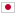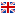Contest Duration: - (local time) (300 minutes) Back to Home
F - Point Distance/Time Limit: 10 sec / Memory Limit: 512 MB

### Problem Statement

You work for invention center as a part time programmer. This center researches movement of protein molecules. It needs how molecules make clusters, so it will calculate distance of all pair molecules and will make a histogram. Molecules’ positions are given by a N x N grid map. Value C_{xy} of cell (x, y) in a grid map means that C_{xy} molecules are in the position (x, y).

You are given a grid map, please calculate histogram of all pair molecules.

### Input

Input is formatted as follows.

N
C_{11} C_{12} ... C_{1N}
C_{21} C_{22} ... C_{2N}
...
C_{N1} C_{N2} ... C_{NN}


First line contains the grid map size N (1 \leq N \leq 1024). Each next N line contains N numbers. Each numbers C_{xy} (0 \leq C_{xy} \leq 9) means the number of molecule in position (x,y). There are at least 2 molecules.

### Output

Output is formatted as follows.

D_{ave}
d_{1} c_{1}
d_{2} c_{2}
...
d_{m} c_{m}


Print D_{ave} which an average distance of all pair molecules on first line. Next, print histogram of all pair distance. Each line contains a distance d_i and the number of pair molecules c_i(0 \lt c_i). The distance d_i should be calculated by squared Euclidean distance and sorted as increasing order. If the number of different distance is more than 10,000, please show the first 10,000 lines. The answer may be printed with an arbitrary number of decimal digits, but may not contain an absolute or relative error greater than or equal to 10^{-8}.

### Sample Input 1

2
1 0
0 1


### Output for the Sample Input 1

1.4142135624
2 1


### Sample Input 2

3
1 1 1
1 1 1
1 1 1


### Output for the Sample Input 2

1.6349751825
1 12
2 8
4 6
5 8
8 2


### Sample Input 3

5
0 1 2 3 4
5 6 7 8 9
1 2 3 2 1
0 0 0 0 0
0 0 0 0 1


### Output for the Sample Input 3

1.8589994382
0 125
1 379
2 232
4 186
5 200
8 27
9 111
10 98
13 21
16 50
17 37
18 6
20 7
25 6


### Source Name

Japan Alumni Group Spring Contest 2013